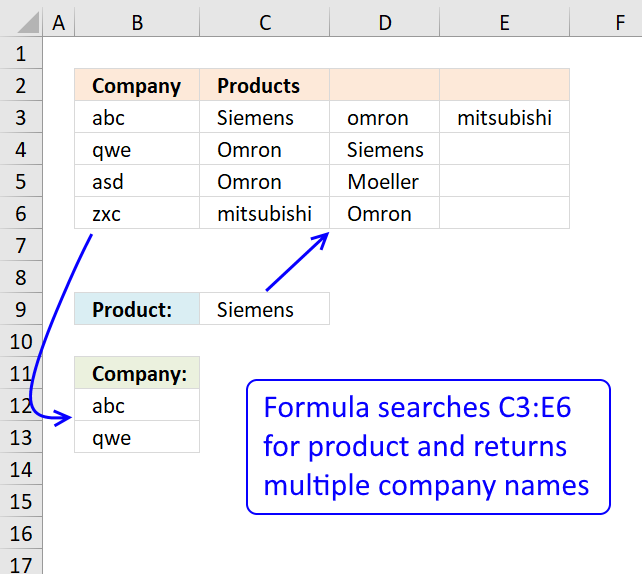Author: Oscar Cronquist Article last updated on January 22, 2019My database is as shown, where I have company abc sells siemens, omron and mitsubishi and company qwe sells omron n siemens.

Company Products
abc Siemens Omron Mitsubishi
qwe Omron Siemens
asd Omron Moeller
zxc Mitsubishi Omron

So right now I wanna key in the product name, lets say siemens, I hope it shows all the companies that sell siemens, in this case would be abc n qwe.

How is it possible? Using your formula can only works for first column, it will shows company abc only (first column), how to make it seach through the second column n show company qwe as well?

This array formula looks up a value in a range (C3:E6) and returns multiple unique distinct values from a column (B3:B6). Cell C9 is the lookup value.

Array formula in cell B12:

=INDEX(\$B\$3:\$B\$6, SMALL(IF((\$C\$3:\$E\$6=\$C\$9)*(COUNTIF(\$B\$11:B11, \$B\$3:\$B\$6)=0), ROW(\$C\$3:\$E\$6)-MIN(ROW(\$C\$3:\$E\$6))+1, ""), 1))

#### Watch a video where I explain how it works

Recommended articles

Recommended articles

VLOOKUP and return multiple values across columns
This article demonstrates a formula that lets you extract non-empty values across columns based on a condition. The image above […]

Recommended articles

Vlookup – Return multiple unique distinct values
Ahmed Ali asks: How to return multiple values using vlookup in excel and removing duplicates? I have tried the formula […]

#### How to create an array formula

1. Double press with left mouse button on cell B12.
2. Copy (Ctrl + c) and paste (Ctrl + v) above array formula to cell B12.
3. Press and hold Ctrl + Shift simultaneously.
4. Press Enter once.
5. Release all keys.

Recommended articles

A beginners guide to Excel array formulas
Array formulas allows you to do advanced calculations not possible with regular formulas.

#### How to copy array formula

1. Select cell B12
2. Copy (Ctrl + c)
3. Select cell range B12:B14
4. Paste ( Ctrl + v)

### Explaining array formula in cell B12

Step 1 - Find matching values in array

(\$C\$3:\$E\$6=\$C\$9)

becomes

{"Siemens", "omron", "mitsubishi";"Omron", "Siemens", 0;"Omron", "Moeller", 0;"mitsubishi", "Omron", 0}="Siemens"

and returns

{TRUE, FALSE, FALSE;FALSE, TRUE, FALSE;FALSE, FALSE, FALSE;FALSE, FALSE, FALSE}

Step 2 - Remove duplicate values in array

COUNTIF(\$B\$11:B11, \$B\$3:\$B\$6)=0

becomes

COUNTIF("Company:", {"abc";"qwe";"asd";"zxc"})=0

becomes

{0; 0; 0; 0}=0

and returns

{TRUE; TRUE; TRUE; TRUE}

Recommended articles

How to use the COUNTIF function
Counts the number of cells that meet a specific condition.

Step 3 - Return row numbers

IF((\$C\$3:\$E\$6=\$C\$9)*(COUNTIF(\$B\$11:B11, \$B\$3:\$B\$6)=0), ROW(\$C\$3:\$E\$6)-MIN(ROW(\$C\$3:\$E\$6))+1, "")

becomes

IF(({TRUE, FALSE, FALSE;FALSE, TRUE, FALSE;FALSE, FALSE, FALSE;FALSE, FALSE, FALSE})*({TRUE; TRUE; TRUE; TRUE}), ROW(\$C\$3:\$E\$6)-MIN(ROW(\$C\$3:\$E\$6))+1, "")

becomes

IF({1, 0, 0;0, 1, 0;0, 0, 0;0, 0, 0}, ROW(\$C\$3:\$E\$6)-MIN(ROW(\$C\$3:\$E\$6))+1, "")

becomes

IF({1, 0, 0;0, 1, 0;0, 0, 0;0, 0, 0}, {1; 2; 3}, "")

and returns

{1, "", "";"", 2, "";"", "", "";"", "", ""}

Recommended articles

How to use the IF function
Checks if a logical expression is met. Returns a specific value if TRUE and another specific value if FALSE.

Step 4 - Find smallest row number

SMALL(IF((\$C\$3:\$E\$6=\$C\$9)*(COUNTIF(\$B\$11:B11, \$B\$3:\$B\$6)=0), ROW(\$C\$3:\$E\$6)-MIN(ROW(\$C\$3:\$E\$6))+1, ""), 1)

becomes

SMALL({1, "", "";"", 2, "";"", "", "";"", "", ""}, 1)

and returns 1.

Recommended articles

How to use the SMALL function
The SMALL function lets you extract a number in a cell range based on how small it is compared to the other numbers in the group.

Step 5 - Return a value or reference of the cell at the intersection of a particular row and column

=INDEX(\$B\$3:\$B\$6, SMALL(IF((\$C\$3:\$E\$6=\$C\$9)*(COUNTIF(\$B\$11:B11, \$B\$3:\$B\$6)=0), ROW(\$C\$3:\$E\$6)-MIN(ROW(\$C\$3:\$E\$6))+1, ""), 1))

becomes

=INDEX(\$B\$3:\$B\$6, 1)

and returns abc in cell B12.

Recommended articles

How to use the INDEX function
Gets a value in a specific cell range based on a row and column number.

### Get the Excel fileVlookup-a-cell-range.xls